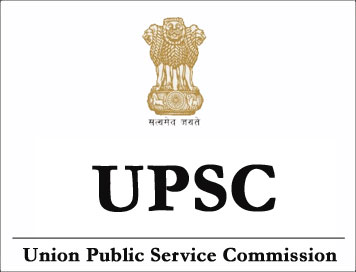NEW!

# (Syllabus) Indian Statistical Service Examination (ISS) - STATISTICS - III## STATISTICS-III

1. Sampling Techniques: Census versus sample survey. Pilot and large scale sample surveys. Role of NSS organisation. Simple random sampling with and without replacement. Stratified sampling and sample allocations. Cos and Variance functions. Ratio and Regression methods of estimation. Sampling with probability proportional to size. Cluster, double, multiphase, multistage and systematic sampling. Interpenetrating sub-sampling. Nonsampling errors.

2. Design and Analysis of Experiments: Principles of design of experiments. Layout and analysis of completely randomised, randomised block and Latin square designs. Factorial experiments and confounding in 2n and 3n experiments. Split-plot and strip-plot designs. Construction and analysis of balanced and partially balanced incomplete block designs. Analysis of covariance. Analysis of non-orthogonal data. analysis of missing and mixed plot data.

3. Economic Statistics: Components of time series. Methods of their determination-variate difference method. Yule-Slutsky effect. Correlogram. Autoregressive models of first and second order. Periodogram analysis. Index numbers of prices and quantities and their relative merits. Construction of index numbers of wholesale and consumer prices. Income distribution- Pareto and Engel curves. Concentration curve. Methods of estimating national income. Inter-sectoral flows. Inter-industry table. Role of CSO.

4. Econometrics: Theory and analysis of consumer demand-specification and estimation of demand functions. Demand elasticities. Structure and model. Estimation of parameters in single equation model-classical least squares, generalised least-square, heteroscedasticity, serial correlation, multi-collinearity, errors in variable model. Simultaneous equation models-Identification, rank and other conditions. Indirect least squares and two stage least squares. Short-term economic forecasting.